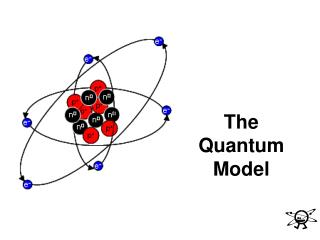DownloadDownload PresentationThe Quantum Model

# The Quantum Model

Download Presentation## The Quantum Model

- - - - - - - - - - - - - - - - - - - - - - - - - - - E N D - - - - - - - - - - - - - - - - - - - - - - - - - - -
##### Presentation Transcript

1. The Quantum Model

2. IThe Quantum Mechanical Model • Erwin Schrodenger (1887-1961) In 1926 Schrodenger proposed our modern model for the atom, the quantum mechanical model, which is based on mathematical calculations.

3. The Quantum Mechanical Model • Like the Bohr model, electrons are restricted to certain energy levels. Unlike the Bohr model, electrons do NOT have to take an exact path around the nucleus.

4. The Quantum Mechanical Model The importance of the quantum mechanical model is that it: • determines allowed energies an electron can have • how likely it is to find the electron in a particular location

5. The Quantum Mechanical Model -We cannot know both the location and velocity of an electron (Heisenberg’s uncertainty principle), thus Schrodinger’s equation does not tell us the exact location of the electron, rather it describes the probability that an electron will be at a certain location in the atom.

6. The Quantum Mechanical Model Heisenberg’s Uncertainty Principle: the exact location and momentum of an object cannot be determined at the same time. If we find the location of an electron, in the process we change its velocity. If we know its velocity, then we have changed its position!!

7. The Quantum Mechanical Model • Today we say that the electrons are located in a region outside the nucleus called the electron cloud. The nucleus is not a little “planet”- rather the way we look at atoms is now like a probability map. Where the cloud makes up regions where electrons may be found.

8. Electron Cloud • Energy Levels Electrons are found in various energy levels around the nucleus. The energy levels are analogous to the rungs of a ladder. The lowest rung of the ladder corresponds to the lowest energy level. A person can climb up or down a ladder by going from rung to rung. Similarly, an electron can jump from one energy level to another. A person on a ladder cannot stand betweenthe rungs, similarly, the electrons in an atom cannot exist between energy levels.

9. A. Quantum • To move from one rung to another, a person climbing a ladder must move just the rightdistance. To move from one energy level to another, an electron must gain or lose just the right amount of energy. The exact amount of energy required to move from one energy level to another is called a quantum of energy.

10. B. Photon • When electrons move from one energy level to another energy level we see light – going from one energy level to another energy level gives off an exact amount of light (called a photon).

11. Quantum Mechanics Model of the Atom • Unlike the rungs of a ladder, the energy levels in an atom are not equally spaced. In fact, the energy levels become more closely spaced the farther they are from the nucleus. Locating an electron in an atom is much like trying to find a person in a hotel.

12. A. Energy Levels- “hotel floor” • energy levels are assigned values in order of increasing energy: n=1,2,3,4, and so forth. • Which energy level is furthest away from the nucleus and has electrons with the highest energy - 1,2,3, or 4? 4

13. B. Sublevels-”type of room” • Within each energy level, the electrons are located in various sublevels – there are 4 different sublevels s, p, d, and f. • Each type of sublevel has a unique shape! Remember we’re talking about what the probability map looks like!

14. C. Orbitals-”beds” Where are the electrons in the various sublevels located in relation to the nucleus? • Electrons areNOTconfined to a fixed circular path, they are, however, found in definite regions of the atoms – these regions are called atomic orbitals! • Each orbital can only hold 2 electrons at a time

15. Within the s sublevel there is only 1 orbital (which is spherical); it is called the s orbital.

16. Within the p sublevel there are 3 orbitals (which are dumbbell shaped) called the p orbitals.

17. Within the d sublevel there are 5 orbitals (4 of which are clover leaf shaped) called the d orbitals.

18. Within the f sublevel there are 7 orbitals - which are too complex to draw or visualize.

19. No let’s draw this “Electron Cloud Hotel” on the back of your notes! 

20. Review “Electron Cloud Hotel” • Energy levels = “floors” • 1-8 • Sublevels = “room shape” • s, p, d, f • Orbitals = “# of beds” • s= 1, p = 3, d= 5, f = 7 • Only 2 electrons in each orbital/bed spinning in opposite direction • s= 2 e-, p = 6 e-, d = 10 e-, f = 14 e-

21. Periodic Patterns!